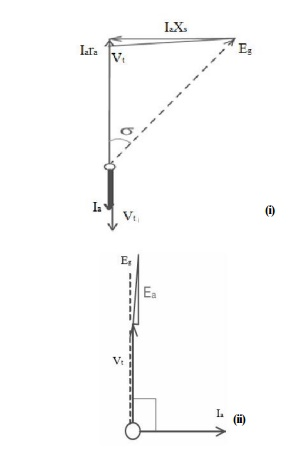Home | | Electrical Machines II | Load Characteristics - Synchronous Generator

# Load Characteristics - Synchronous Generator

Consider a synchronous generator driven at constant speed and with constant excitation.

Consider a synchronous generator driven at constant speed and with constant excitation. On open circuit the terminal voltage Vt is the same as the open circuit e.m.f. Eg. Suppose a unity-power-factor load be connected to the machine. The flow of load current produces a voltage drop IaZs in the synchronous impedance, and terminal voltage Vt is reduced. Fig. 1.12 shows the phasor diagram for three types of load. It will be seen that the angle between Eg and Vt increases with load, indicating a shift of the flux across the pole faces due to cross- magnetization. The terminal voltage is obtained from the complex summationso that the V/I curve, Fig. 1.13, is nearly an ellipse with semi-axes Eg and Isc. The current Isc is that which flows when the load resistance is reduced to zero. The voltage Vt falls to zero also and the machine is on short-circuit with Vt = 0 and

Ia =Isc =Eg /Zs

~  Eg /Xs

For a lagging load of zero power-factor, diagram is given in Fig. 1.13. The voltage is given as before and since the resistance in normal machines is small compared with the synchronous reactance, the voltage is given approximately bywhich is the straight line marked for cosϕ = 0 lagging in Fig.1.14. A leading load of zero power factor Fig. 1.14 will have the voltageanother straight line for which, by reason of the direct magnetizing effect of leading currents, the voltage increases with load.

Intermediate load power factors produce voltage/current characteristics resembling those in Fig: 1.13 The voltage-drop with load (i.e. the regulation) is clearly dependent upon the power factor of the load. The short-circuit current Isc at which the load terminal voltage falls to zero may be about 150 per cent (1.5 per unit) of normal current in large modern machines.Study Material, Lecturing Notes, Assignment, Reference, Wiki description explanation, brief detail
Electrical machines : Synchronous Generator : Load Characteristics - Synchronous Generator |